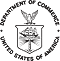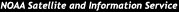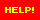formerly the National Oceanographic Data Center (NODC)...  more on NCEIThis Site All of NOAA

NCEI is transitioning to a new website and paths to data resources will be changing. Please contact NCEI.Info@noaa.gov with any questions of issues. See the new website at www.ncei.noaa.gov.

 You are here: NODC Home  > Interactive Data Access and Retrieval System  > Unit Conversion Guide

# Interactive Data Access and Retrieval System

Coastal Water Temperature Guide
Global Argo Data Repository
Global Temperature-Salinity Profile Program
NOAA OI V2 Sea Surface Temperatures
Animation of Monthly-averaged Sea Surface Temperatures
Global Ocean Currents Database
Tools
Unit Conversion Guide
details at right»

>> Unit Conversion Guide

 This site uses Java and JavaScript programming languages for making pages interactive. To view this site, please have Java and JavaScript enabled. Note: How to use the conversion factors: Enter a number in either box, then click the other box. IMPORTANT NOTICE: Do not use the figures given here as sole source for any commercial (or legal) purpose involving cost, money, sales, purchase etc. Any consequences thereof are the sole responsibility of the person doing the calculation.
 To convert Into Multiply by Examples: square meters (m²) square centimeters (cm²) 10000 m² = cm² square meters (m²) square feet (ft²) 10.763911 m² = ft² square kilometers (km²) (statute) square miles (mi²) 0.386109 km²= mi² square kilometers (km²) nautical square miles (nm²) 0.291181 km²= nm² square kilometers (km²) acres 247.105381 km²= acres (statute) square miles (mi²) acres 640.00 mi²= acres acres square yards (yd²) 4840 acres= yd² acres square feet (ft²) 43560 acres= ft² hectares(ha) acres 2.47105381 hectares= acresTo convert Into Multiply by Examples: meters (m) centimeters (cm) 100 m= cm meters (m) inches (in) 39.37008 m= in meters (m) feet (ft) 3.280840 m= ft meters (m) yard (yd) 1.093613 m= yd kilometers (km) (statute) miles (mi) 0.621371 km= mi kilometers (km) nautical miles (nm) 0.539612 km= nm feet (ft) inches (in) 12.0 ft= inTo convert Into Multiply by Examples: kilograms (kg) grams (g) 1000 kg= g kilograms (kg) pounds (lb) 2.204627 kg= lb grams (g) ounce (oz) 0.035274 g= ozPressure

To convert

Into

Multiply by

Examples:

atmospheres (atm) millibar (mb) 1013.25 atm= mb
atmospheres (atm) feet of water (at 4°C) 33.90 atm= ftof water
atmospheres (atm) inches of mercury (at 0°C) 29.92 atm= in. of mercury
atmospheres (atm)  centimeters of mercury 76.0 atm= cmsof mercury
atmospheres (atm)  kgs/cm² 1.0333 atm= kgs/cm²
atmospheres (atm)  lbs/in² 14.70 atm= lbs/in²
atmospheres (atm)  tons/ft² 1.058 atm= tons/ft²To convert Into Multiply by Examples: kilometers/hour (km/h) meters/second (m/s) 0.277778 km/h= m/s kilometers/hour (km/h) miles/hour (mi/hr) 0.6214 km/h= mi/hr knots(kn) meters/second (m/s) 0.514444 kn= m/sTo convert Into Multiply by Examples: Fahrenheit (°F)-32 Celsius (°C) 5/9 °F= °C Fahrenheit (°F)+459.67 kelvin (K) 5/9 °F= K Celsius (°C)+17.7778 Fahrenheit (°F) 1.8 °C= °F Celsius (°C)+273.15 kelvin (K) 1 °C= KTo convert Into Multiply by Examples: cubic meters (m³) cubic centimeters (cm³) 1,000,000 m³ = cm³ cubic meters (m³) cubic feet (ft³) 35.31467 m³ = ft³ cubic meters (m³) U.S. gallons (gal) 264.1721 m³= gal liter(l) U.S. gallons (gal) 0.2641721 liter= gal